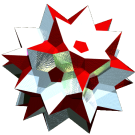Acronym tikko Name triakis octahedron,apiculated octahedron© Inradius sqrt[(23+16 sqrt(2))/68] = 0.819141 Vertex figure [t8], [T3] Coordinates (1/2, 1/2, 1/2)             & all permutations, all changes of sign (vertex inscribed cube) ((1+sqrt(2))/2, 0, 0)    & all permutations, all changes of sign (vertex inscribed (2+sqrt(2))/2-oct) Dihedral angles at long edge:   arccos[-(3+8 sqrt(2))/17] = 147.350100° at short edge:   arccos[-(3+8 sqrt(2))/17] = 147.350100° Dual tic ExternallinksThe triangles {(t,t,T)} have vertex angles t = arccos[(2+sqrt(2))/4] = 31.399715° resp. T = arccos[-(2 sqrt(2)-1)/4] = 117.200570°.

Edge sizes used here are tT = x = 1 (short) resp. tt = a = (2+sqrt(2))/2 = 1.707107 (long).

This polyhedron also can be obtained as the hull of a central cube with 6 attached squippies.

Incidence matrix according to Dynkin symbol

o3m4m =
ao3oo4ox&#zx   → height = 0
a = (2+sqrt(2))/2 = 1.707107

o.3o.4o.    | 6 * |  4  4 |  8  [t8]
.o3.o4.o    | * 8 |  0  3 |  3  [T3]
------------+-----+-------+---
a. .. ..    | 2 0 | 12  * |  2  a
oo3oo4oo&#x | 1 1 |  * 24 |  2  x
------------+-----+-------+---
ao .. ..&#x | 2 1 |  1  2 | 24  {(t,t,T)}